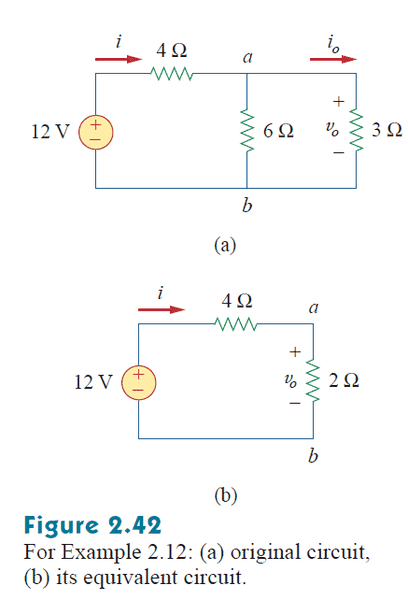Confusion about combining resistors in series.

Hello, everybody.

I'm reading through my EE textbook and I'm having some confusion about when to combine resistors in series. The following figure is in my book:I see that they combine the parallel resistors of 6Ω and 3Ω to make the equivalent circuit shown in 2.42b. What I'm confused about is why they didn't then combine the 4Ω and 2Ω resistors. They do this throughout the entire chapter's examples and it's causing me confusion. Resistors are considered in series if they share the same current, correct? And the two resistors in 2.42b appear to share the same current, so I don't see why they don't combine them.

(I apologize if this is considered homework help. It seems to be more of a general circuit question.)

vk6kro
If you only wanted to get the current from the battery, you would combine all the resistors.

However, if you wanted to get the voltage across the parallel comination, then you would have to keep that resistance separate so that you could calculate the voltage.

In fact you could do both. The combined resistance is 6 ohms (4 ohms + 2 ohms) so the current is 2 amps (12 volts / 6 ohms).

So the voltage across (a) - (b) is 4 volts. (V = i * R = 2amps * 2 ohms = 4 volts)

sophiecentaur